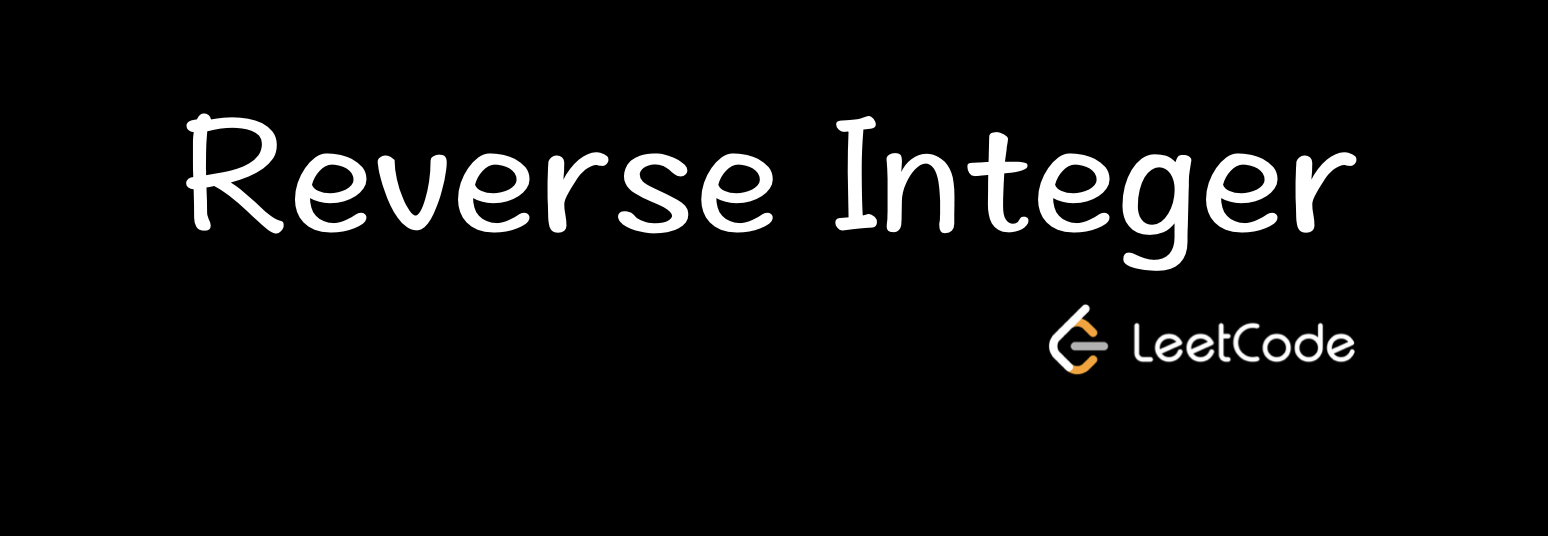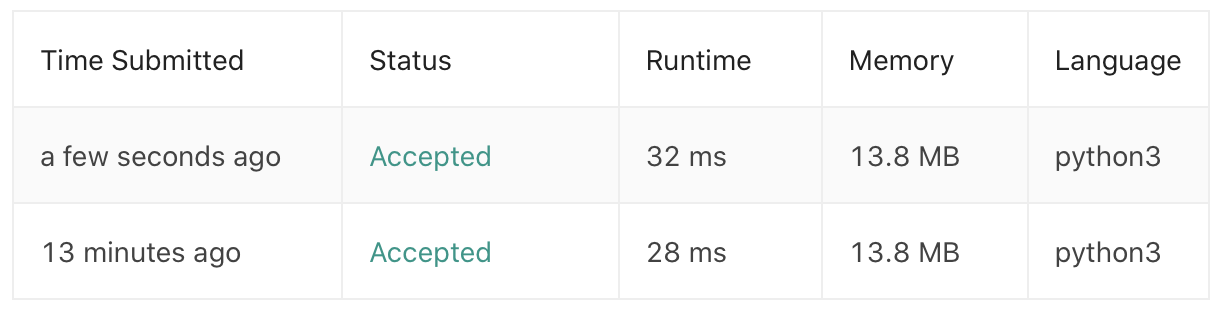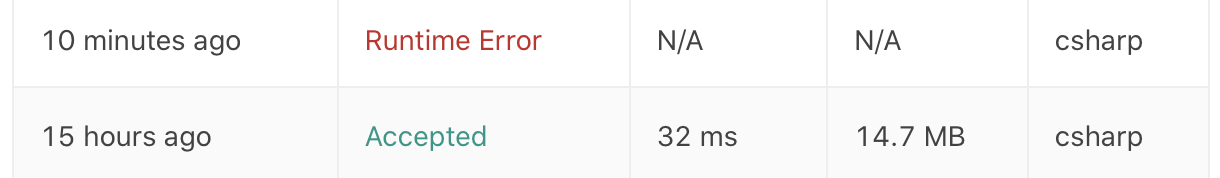# LeetCode | 2. Reverse Integer 整数反转PuranReverse Integer 是 LeetCode 算法题库中的第七道题，难度为 Easy，题目地址为：https://leetcode.com/problems/reverse-integer/

## 1. 问题描述

Input 123Output 321﻿Input -123Output -321

﻿

## 2. 解题思路

1. 转换为字符串：这个思路是我一开始看到该问题，就自然想到的一个方法。将整数转化为字符串，再通过字符串的反转来求解。

2. 借用堆栈的 Pop/Push：该方法是看到官方提供的 Solution 才知道的，即类似于采用堆栈的先进先出的概念，对整数本身进行处理，对原始数值依次进行弹出（Pop）操作，在反转的数值中将之前弹出的数值进行推入（Push）操作。

﻿

﻿

## 3. 知识点

### Python

• Python 的列表及其切片

• Python 的取模操作

• Python 的 int 最大值

#### 列表及其切片

Python 中的列表就相当于数组，可以通过下标来取得列表中的单个值。虽然下标hi从0开始向上增长的，但也可以通过负整数作为下标。比如，-1是指列表中的最后一个下标，-2是指列表中倒数第二个下标。

list

list[0:2]list[:]

L = range(10)L[::2] //range(0, 10, 2)L[::-1] //range(9, -1, -1)

﻿

#### Python 的取模操作

1. 求整数商：c = a / b

2. 取模或求余：r = a - c * b

• 求余操作时，计算 c 的值是向 0 方向舍入

• 取模操作时，计算 c 的值是向负无穷方向舍入

﻿

﻿

•  -7 % 4 = 1 为例

首先，计算-7 / 4 = 1.75，在取模操作时，需向负无穷方向舍入，因此 c = -2；由此得到最终的结果为：r = -7 - (-2 * 4) = 1。

﻿

•  7 % -4 = -1 为例

首先，计算 7 / -4 = -1.75，在取模操作时，依旧是向负无穷方向舍入，因此 c = -2；由此得到最终的结果为：r = 7 - (-2 * -4) = -1。

﻿

﻿

﻿

## 4. 代码

﻿

### Python 实现

class Solution:    def reverse(self, x: int) -> int:        maxint = 2**31 - 1        minint = -2**31        flag = 1        if x < 0:            flag = -1        x = x * flag        x_str = str(x)        reverse = int(x_str[::-1]) * flag        if (reverse > maxint or reverse < minint):            return 0        return reverse

﻿

Pop/Push 方法实现：

class Solution:    def reverse(self, x: int) -> int:        reverse = 0        maxint = 2**31 - 1        minint = -2**31        if x > 0:            while (x != 0):                pop = x % 10                x = int(x/10)                if (reverse > maxint/10 or (reverse == maxint/10 and pop > 7)):                    return 0                reverse = reverse * 10 + pop        elif x < 0:            while(x != 0):                pop = -(-x % 10)                x = int(x/10)                if (reverse < minint/10 or (reverse == minint/10 and pop < -8)):                    return 0                reverse = reverse * 10 + pop        return reverse

﻿﻿

### C# 实现

﻿

Pop/Push 的实现：

public class Solution {    public int Reverse(int x) {        int reverse = 0;        int maxint = Int32.MaxValue;        int minint = Int32.MinValue;        while (x != 0)        {            int pop = x % 10;            x = x/10;            if (reverse > maxint/10 || (reverse == maxint/10 && pop > 7))            {                return 0;            }            if (reverse < minint/10 || (reverse == minint/10 && pop < -8))            {                return 0;            }            reverse = reverse * 10 + pop;        }        return reverse;    }}

﻿

public class Solution {    public int Reverse(int x) {        int reverse = 0;        int maxint = Int32.MaxValue;        int minint = Int32.MinValue;        int flag = 1;        if (x < 0)        {            flag = -1;        }        x = x * flag;        string x_str = x.ToString();        char[] x_str_Array = x_str.ToCharArray();        Array.Reverse(x_str_Array);        string reverse_string = new string(x_str_Array);        double value = Double.Parse(reverse_string);        if (value > maxint || value < minint)        {            return 0;        }        else         {            reverse = Int32.Parse(reverse_string) * flag;            return reverse;        }    }}

﻿﻿### Puran

GIS从业者，正在往开发的路上小跑。 2018.03.29 加入

## 评论